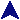InheritanceRuby Example Code# Class names must be capitalized.  Technically, it's a constant.
class Fred

# The initialize method is the constructor.  The @val is
# an object value.
def initialize(v)
@val = v
end

# Set it and get it.
def set(v)
@val = v
end

def get
return @val
end

def more(y)
@val += y
end

def less(y)
@val -= y
end

def to_s
return "Fred(val=" + @val.to_s + ")"
end
end

# Class Barney is derived from Fred with the usual meaning.
class Barney < Fred
def initialize(x)
super(x)
@save = x
end

def chk
@save = @val
end

def restore
@val = @save
end

def to_s
return "(Backed-up) " + super + " [backup value: " + @save.to_s + "]"
end

end

# Objects are created by the new method of the class object.
a = Fred.new(398)
b = Barney.new(112)

a.more(34)
b.more(817)

print "A: a = ", a, "\n   b = ", b, "\n";

a.more(34)
b.more(817)

print "B: a = ", a, "\n   b = ", b, "\n";

b.chk

a.more(34)
b.more(817)

print "C: a = ", a, "\n   b = ", b, "\n";

b.restore

print "D: a = ", a, "\n   b = ", b, "\n";